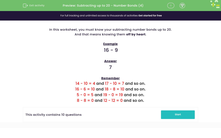# Practise Simple Subtraction Skills Including Number Bonds Up to 20

In this worksheet, students will be tested on their subtraction number bonds up to 20.Key stage:  KS 1

Curriculum topic:   Number: Addition and Subtraction

Curriculum subtopic:   Add and Subtract to 20

Difficulty level:#### Worksheet Overview

In this activity, there will be a mixture of questions. There will be some subtraction number bonds that you will need to know by heart, and some simple subtraction questions that you will need to work out.

We should know the subtracting number bonds to 20 off by heart.

The stars will remind us of the subtracting number bonds to 10:We can see that the subtraction facts are the same as the addition facts but just the other way around.

For example, 10 - 1 = 9 would be 9 + 1 = 10. The numbers just get moved around, so if you know your addition number bonds, you will know your subtraction bonds!

Now, let's look at the number bond subtraction facts to 20.

20 - 0 = 20

20 - 1 = 19

20 - 2 = 18

20 - 3 = 17

20 - 4 = 16

20 - 5 = 15

20 - 6 = 14

20 - 7 = 13

20 - 8 = 12

20 - 9 = 11

20 - 10 = 10

Can you follow the pattern to complete all the subtraction number bonds to 20?

Example 1

20 - _ = 5

If you are able to work out the missing number in this subtraction number bond off by heart, then brilliant, just write in the answer.

If you are not sure, move it around into an addition number bond:

5 + _  = 20

What would go with 5 to make 20? It's 15. Remember, if you know your addition number bonds, you will know your subtraction number bonds!

Example 2

14 - 7 =

Remember, with subtraction we can't move the numbers around, the biggest number must always be first.

You may be able to count backwards to work out the answer, or you can count forwards and find the difference. We need to jump up from 7 to 14.

We could also use our number bonds and jump up to 10 and then jump to 14 like this:So, we add on 3 to get to 10, and then we add on 4 to get to 14. We have added 3 and 4, so we have added 7 altogether. This gives us the answer of 7.

We don't need to draw number lines, but we could work out the answers using this technique in our heads!Are you ready to have a go?

### What is EdPlace?

We're your National Curriculum aligned online education content provider helping each child succeed in English, maths and science from year 1 to GCSE. With an EdPlace account you’ll be able to track and measure progress, helping each child achieve their best. We build confidence and attainment by personalising each child’s learning at a level that suits them.

Get started